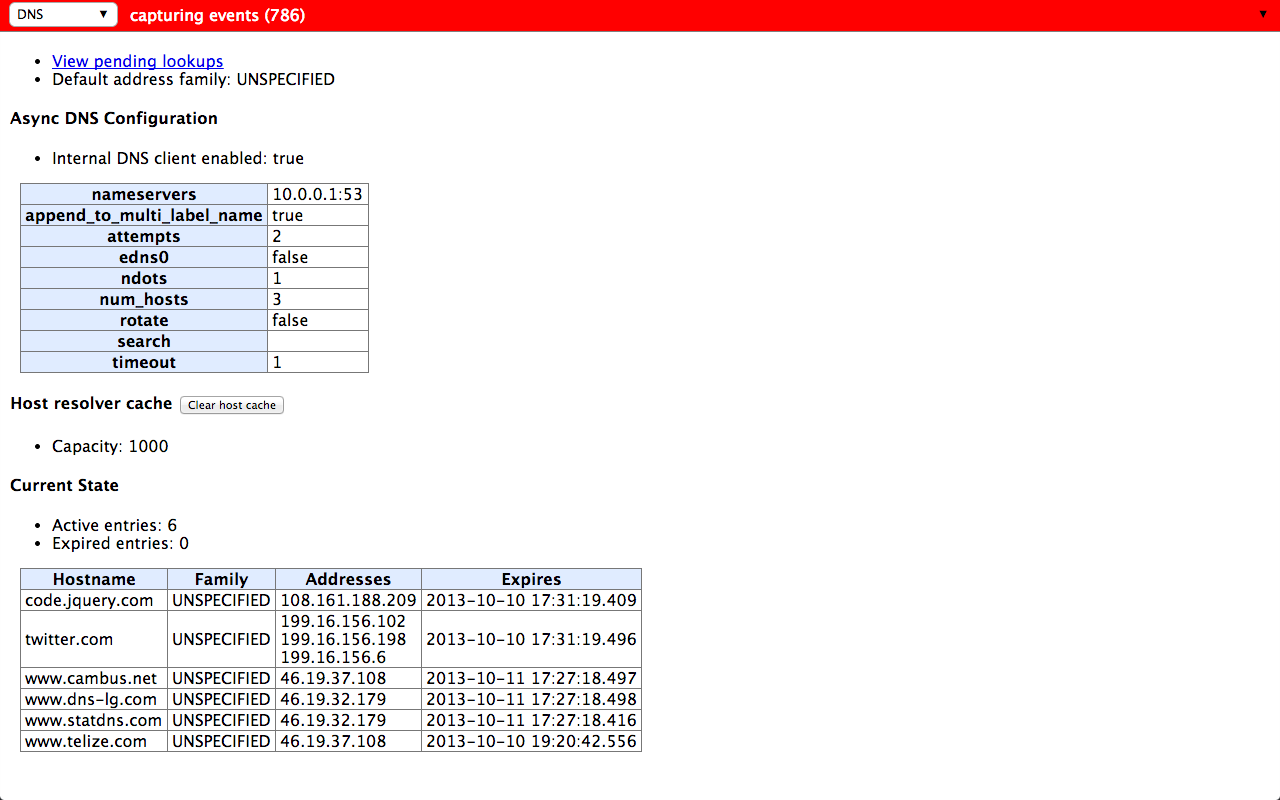# Inspecting DNS Prefetching and resolver performance within Chrome

Frederic Cambus October 11, 2013 [DNS]

With Chrome and Firefox both performing DNS prefetching, it can be useful to inspect and understand what's happening behind the scenes when the process takes place. Fortunately, Chrome has some really nice internal tools which can be used for this purpose.

### Using net-internals to visualize DNS prefetching

Pointing Chrome to chrome://net-internals/#dns will start running a visualizer for the NetLog (Chrome's internal network logging system) DNS event stream.

After clearing the host resolver cache, we can load a new page and get a list of all hosts that were queried.### Inspecting resolver performance using Chrome histograms

Pointing Chrome to chrome://histograms/DNS will display metrics about various DNS performance measurements.

A particularly interesting one is "AsyncDNS.ResolveSuccess", representing the distribution of resolution time (in milliseconds):

``````Histogram: AsyncDNS.ResolveSuccess recorded 3732 samples, average = 263.9 (flags = 0x1)
0     ...
2     -O                                                                        (3 = 0.1%) {0.0%}
3     --O                                                                       (5 = 0.1%) {0.1%}
4     ---O                                                                      (6 = 0.2%) {0.2%}
5     -O                                                                        (3 = 0.1%) {0.4%}
6     O                                                                         (0 = 0.0%) {0.5%}
7     O                                                                         (1 = 0.0%) {0.5%}
8     O                                                                         (0 = 0.0%) {0.5%}
9     O                                                                         (1 = 0.0%) {0.5%}
10    O                                                                         (0 = 0.0%) {0.5%}
12    O                                                                         (1 = 0.0%) {0.5%}
14    ...
24    O                                                                         (1 = 0.0%) {0.5%}
28    O                                                                         (3 = 0.1%) {0.6%}
32    O                                                                         (1 = 0.0%) {0.6%}
37    O                                                                         (4 = 0.1%) {0.7%}
43    ---O                                                                      (32 = 0.9%) {0.8%}
50    -----O                                                                    (57 = 1.5%) {1.6%}
58    ------------O                                                             (134 = 3.6%) {3.2%}
67    ----------O                                                               (115 = 3.1%) {6.8%}
77    --------O                                                                 (85 = 2.3%) {9.8%}
89    ------------------------------------O                                     (405 = 10.9%) {12.1%}
103   ------------------------------------------------------------------------O (808 = 21.7%) {23.0%}
119   ------------------------------------O                                     (403 = 10.8%) {44.6%}
137   -----------O                                                              (125 = 3.3%) {55.4%}
158   ------------O                                                             (130 = 3.5%) {58.8%}
182   -------------O                                                            (146 = 3.9%) {62.2%}
210   ---------O                                                                (106 = 2.8%) {66.2%}
242   --------O                                                                 (95 = 2.5%) {69.0%}
279   -----------O                                                              (121 = 3.2%) {71.5%}
322   ----------O                                                               (109 = 2.9%) {74.8%}
372   ----------O                                                               (109 = 2.9%) {77.7%}
429   -----------O                                                              (124 = 3.3%) {80.6%}
495   ------------O                                                             (130 = 3.5%) {83.9%}
571   ---------O                                                                (106 = 2.8%) {87.4%}
659   ---------O                                                                (106 = 2.8%) {90.3%}
761   --------O                                                                 (89 = 2.4%) {93.1%}
878   ----O                                                                     (47 = 1.3%) {95.5%}
1013  -----O                                                                    (59 = 1.6%) {96.8%}
1169  ---O                                                                      (32 = 0.9%) {98.3%}
1349  -O                                                                        (12 = 0.3%) {99.2%}
1557  -O                                                                        (7 = 0.2%) {99.5%}
1797  O                                                                         (3 = 0.1%) {99.7%}
2074  O                                                                         (3 = 0.1%) {99.8%}
2394  O                                                                         (4 = 0.1%) {99.9%}
2763  O                                                                         (0 = 0.0%) {100.0%}
3189  O                                                                         (1 = 0.0%) {100.0%}
3681  ...
``````

Another one is "DNS.PrefetchResolution":

``````Histogram: DNS.PrefetchResolution recorded 1658 samples, average = 333.0 (flags = 0x1)
0      O                                                                         (0 = 0.0%)
15     -O                                                                        (1 = 0.1%) {0.0%}
17     O                                                                         (0 = 0.0%) {0.1%}
19     -O                                                                        (2 = 0.1%) {0.1%}
21     ...
26     O                                                                         (1 = 0.1%) {0.2%}
29     -O                                                                        (3 = 0.2%) {0.2%}
32     O                                                                         (0 = 0.0%) {0.4%}
36     -O                                                                        (2 = 0.1%) {0.4%}
40     --O                                                                       (6 = 0.4%) {0.5%}
45     --O                                                                       (8 = 0.5%) {0.9%}
50     --O                                                                       (9 = 0.5%) {1.4%}
56     -----O                                                                    (19 = 1.1%) {1.9%}
63     ---------O                                                                (34 = 2.1%) {3.1%}
71     --------O                                                                 (30 = 1.8%) {5.1%}
79     -------O                                                                  (27 = 1.6%) {6.9%}
88     -------------------------O                                                (94 = 5.7%) {8.6%}
98     ------------------------------------------------------------------------O (276 = 16.6%) {14.2%}
110    ---------------------------------------------------------O                (218 = 13.1%) {30.9%}
123    --------------------------O                                               (98 = 5.9%) {44.0%}
138    -------------O                                                            (48 = 2.9%) {49.9%}
154    -------O                                                                  (27 = 1.6%) {52.8%}
172    -------O                                                                  (26 = 1.6%) {54.5%}
193    ---------O                                                                (35 = 2.1%) {56.0%}
216    -------------O                                                            (50 = 3.0%) {58.1%}
242    ------------O                                                             (46 = 2.8%) {61.2%}
271    --------------O                                                           (53 = 3.2%) {63.9%}
303    -------------O                                                            (48 = 2.9%) {67.1%}
339    ---------O                                                                (36 = 2.2%) {70.0%}
379    -----------O                                                              (41 = 2.5%) {72.2%}
424    ----------------O                                                         (63 = 3.8%) {74.7%}
475    -----------O                                                              (43 = 2.6%) {78.5%}
532    ---------------O                                                          (57 = 3.4%) {81.1%}
595    ----------O                                                               (40 = 2.4%) {84.5%}
666    -------------O                                                            (49 = 3.0%) {86.9%}
745    -----------O                                                              (43 = 2.6%) {89.9%}
834    --------O                                                                 (32 = 1.9%) {92.5%}
933    ------O                                                                   (24 = 1.4%) {94.4%}
1044   --------O                                                                 (29 = 1.7%) {95.8%}
1168   ----O                                                                     (15 = 0.9%) {97.6%}
1307   -O                                                                        (4 = 0.2%) {98.5%}
1463   -O                                                                        (3 = 0.2%) {98.7%}
1637   -O                                                                        (3 = 0.2%) {98.9%}
1832   -O                                                                        (2 = 0.1%) {99.1%}
2050   O                                                                         (1 = 0.1%) {99.2%}
2295   -O                                                                        (2 = 0.1%) {99.3%}
2569   -O                                                                        (4 = 0.2%) {99.4%}
2875   O                                                                         (0 = 0.0%) {99.6%}
3218   O                                                                         (1 = 0.1%) {99.6%}
3602   O                                                                         (1 = 0.1%) {99.7%}
4032   ...
5051   O                                                                         (1 = 0.1%) {99.8%}
5653   -O                                                                        (2 = 0.1%) {99.8%}
6327   ...
19524  O                                                                         (1 = 0.1%) {99.9%}
21852  ...
``````

There are a lot of DNS performance histograms to explore, and the best thing is that they are displayed in ASCII, making it easy to save, compare, and analyze them.